Learn US

# Congruency of Triangles

963 views

 1 Introduction 2 What are Congruent Triangles? 3 Theorems of Congruency 4 Examples 5 Exercise Questions 6 Summary 7 FAQs 8 External References

14 December 2020

## Introduction

Congruence is defined as agreement or harmony. In this blog, we will understand how to use the properties of triangles, to prove congruency between $$2$$ or more separate triangles. Two triangles are said to be congruent if one can be superimposed on the other such that each vertex and each side lie exactly on top of the other.### Congruency of Triangles-PDF

Learn about the Congruency of Triangles, the different Tests/Theorems to prove congruency of triangles, with Examples. Here is a downloadable PDF to explore more.

## What are congruent triangles?

### Definition

Look at ΔABC and ΔPQR below.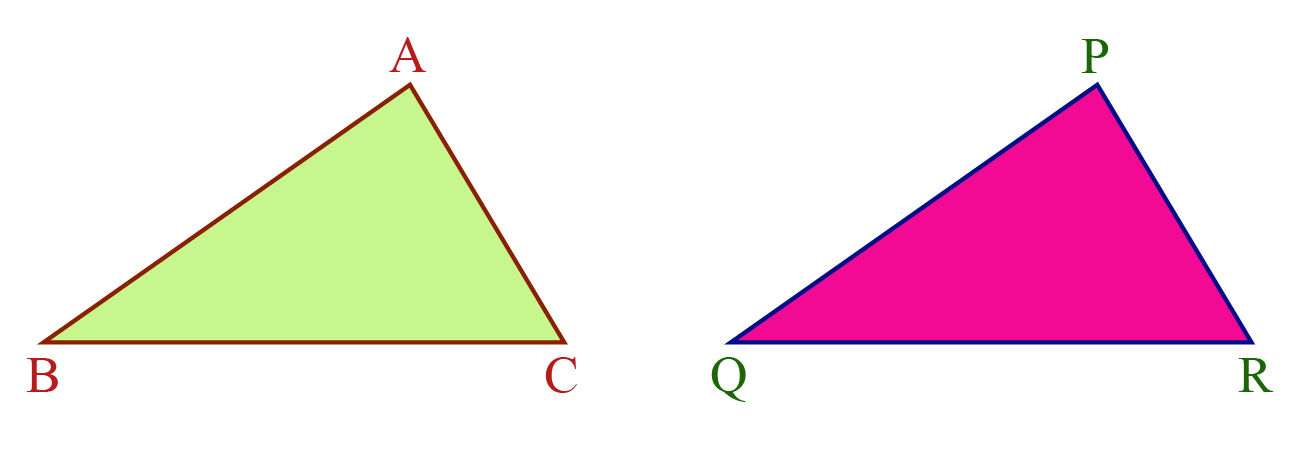These two triangles are of the same size and shape.

Thus, we can say that they are congruent.

Two triangles are said to be congruent if all $$3$$ of their angles and all $$3$$ of their sides are equal. Although these are $$6$$ parameters, we only need $$3$$ to prove congruency.

## Theorems of Congruency

### SSS Postulate (side side side)

If the three sides of a triangle are equal to three sides on another triangle, both triangles are said to be congruent by SSS postulate (Side, Side, Side).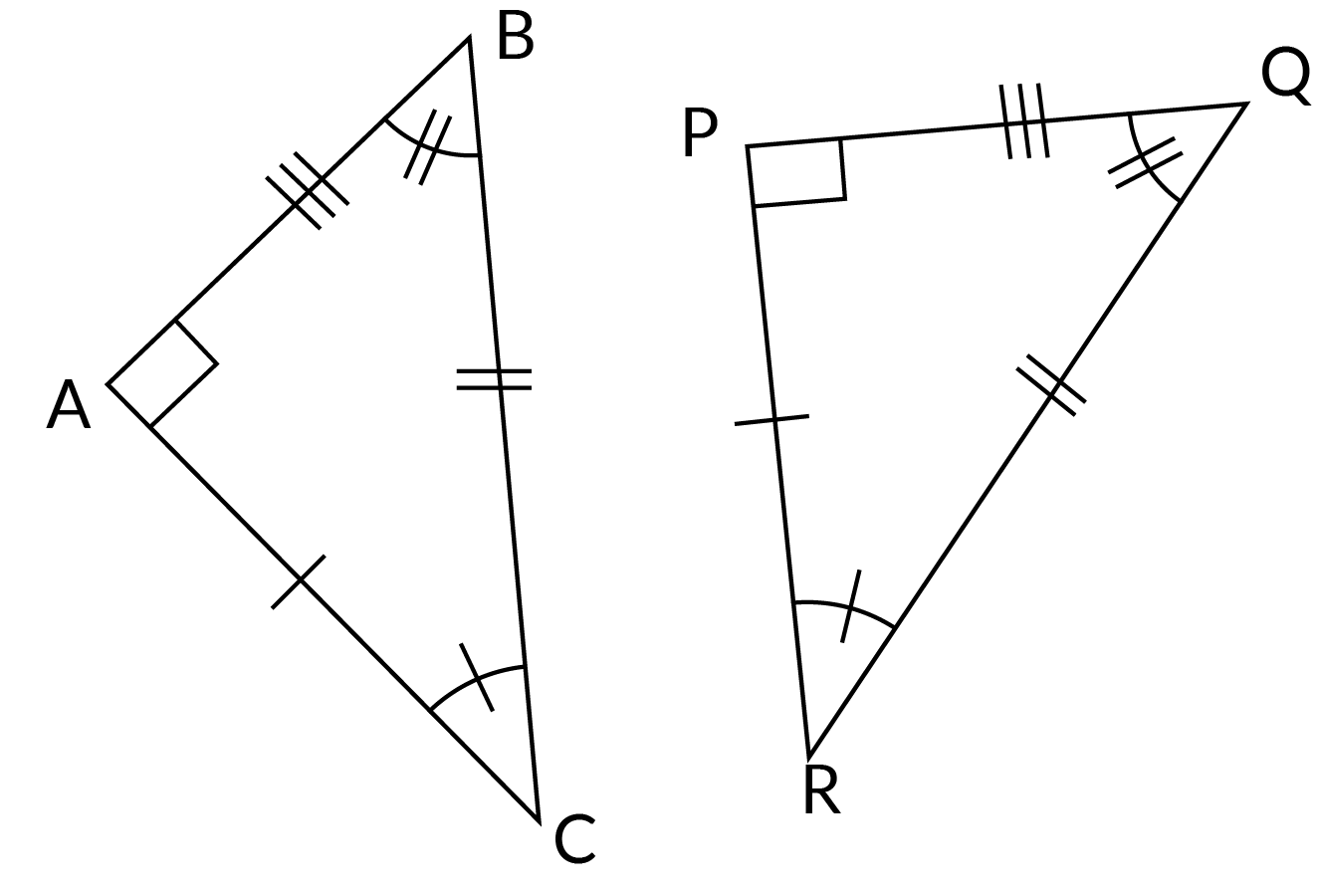### SAS Postulate (side angle side)

If two triangles have one angle equal, and two sides on either side of the angle equal, the triangles are congruent by SAS Postulate (Side, Angle, Side).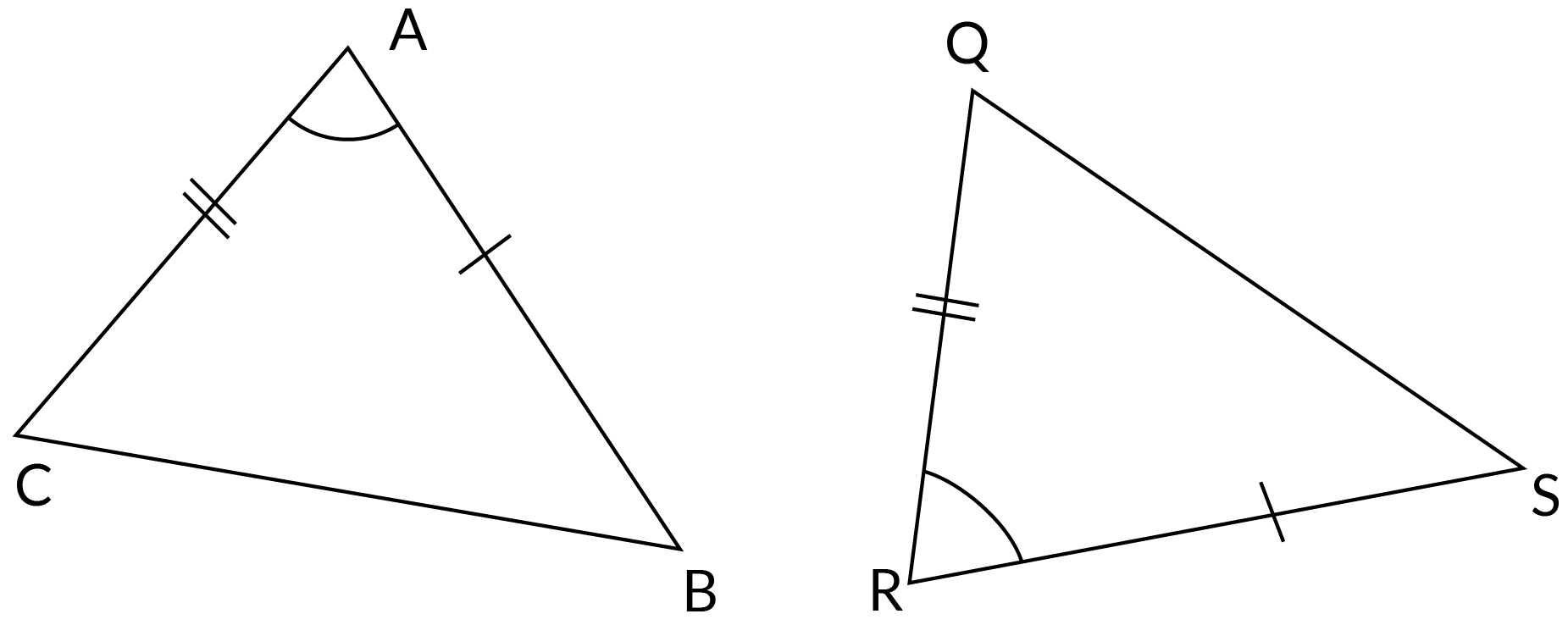### AAS Postulate (angle angle side)

Two equal angles and a side that does not lie between the two angles, prove that a pair of triangles are congruent by the AAS Postulate (Angle, Angle, Side).

### ASA Postulate (angle side angle)

When two angles and a side between the two angles are equal, for $$2$$ triangles, they are said to be congruent by the ASA postulate (Angle, Side, Angle).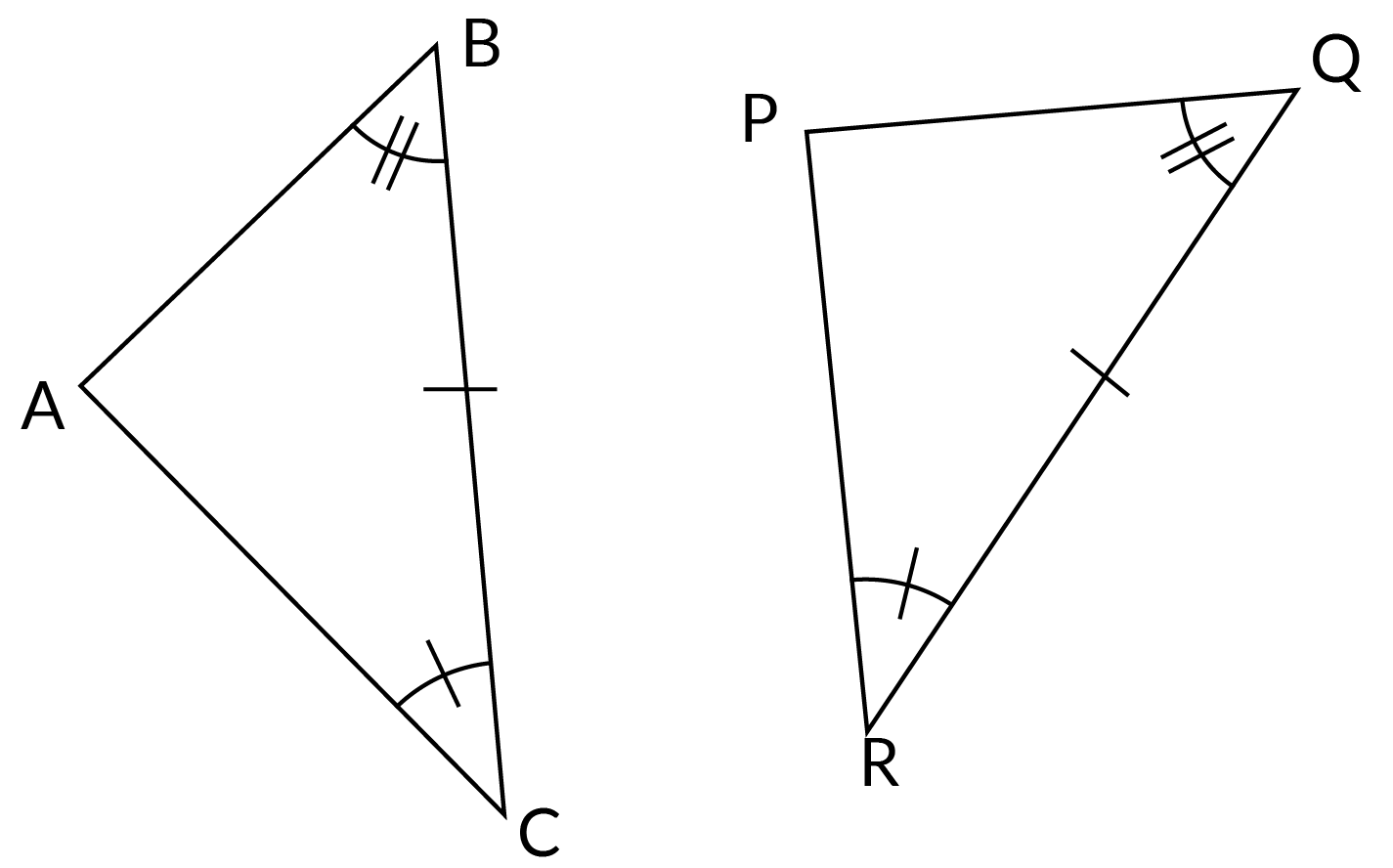### RHS Postulate (Right Angle Hypotenuse Side)

The RHS postulate (Right Angle, Hypotenuse, Side) applies only to Right-Angled Triangles. If the Hypotenuse and a side are equal, then the triangles are congruent.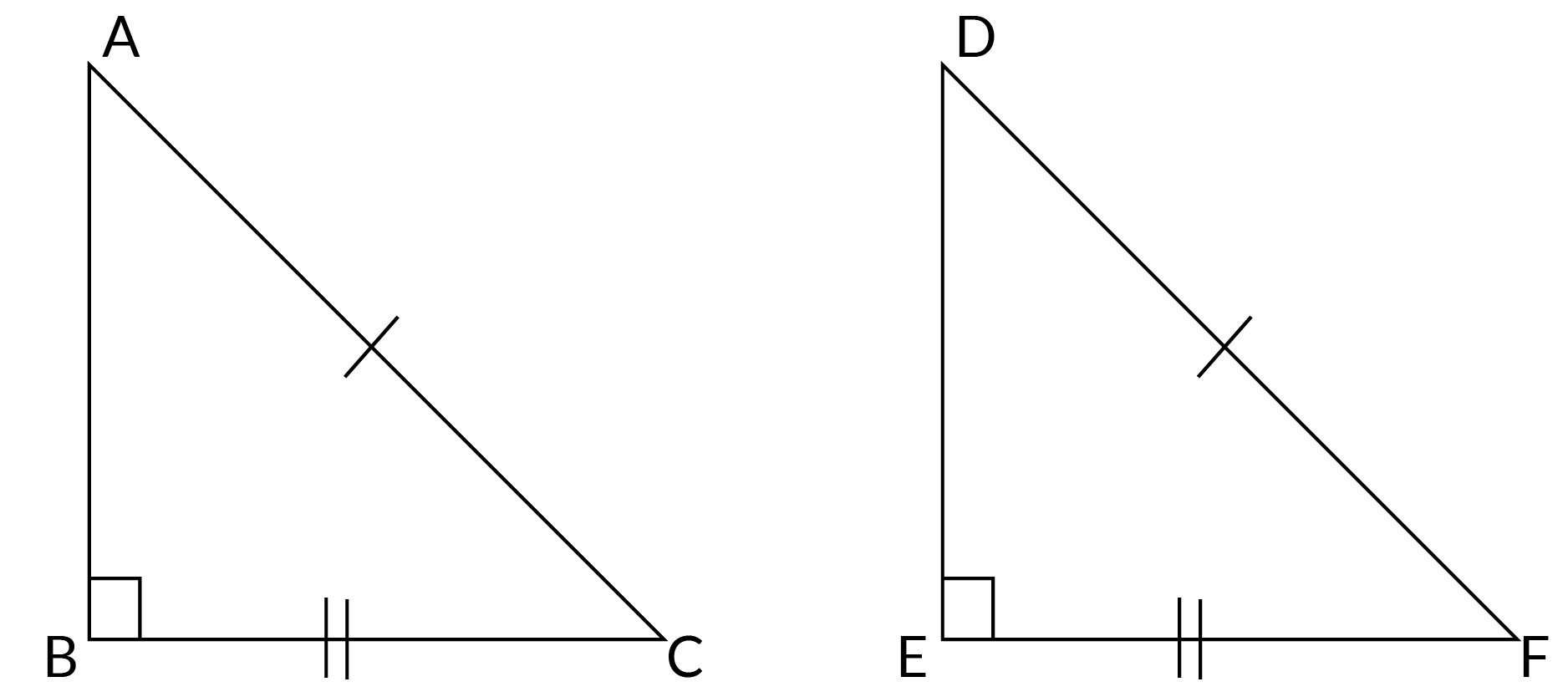### CPCTC

CPCTC is the theorem that states Congruent Parts of a Congruent Triangle are Congruent.

This implies that if two triangles are proven to be congruent, then their corresponding sides and angles are all equal.

## Examples

1. If $$\rm{ABCD}$$ is a parallelogram and $$\rm{AC}$$ is one of its diagonals. What can you say about triangles $$\rm{ABC}$$ and $$\rm{CDA}?$$ Explain your answer.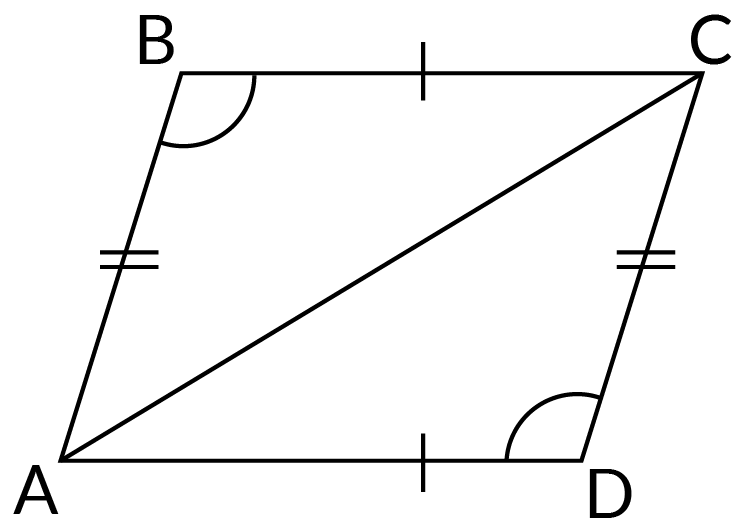Statement Reason
In  $$▱\text{ABCD}$$,
$$\rm{AB} = \rm{CD}$$
Opposite sides of a parallelogram are equal
$$\text{BC} = \text{AD}$$
$$\rm{AC} = \text{AC}$$  Common side
$$\therefore 4\;\triangle \text{ABC} ⩭ \triangle \text{ACD}$$  By SSS Postulate

Hence $$△\rm{ABC}$$ and $$△\rm{ACD}$$ are proved to be congruent.

2. $$\rm{BB}'$$ is the angle bisector of $$∠\rm{ABC}.$$ $$\rm{ABC}$$ is an isosceles triangle. Show that triangles $$\rm{ABB}'$$ and $$\rm{CBB}'$$ are congruent. What is the relation between $$\rm{AB}’$$ and $$\rm{CB}’$$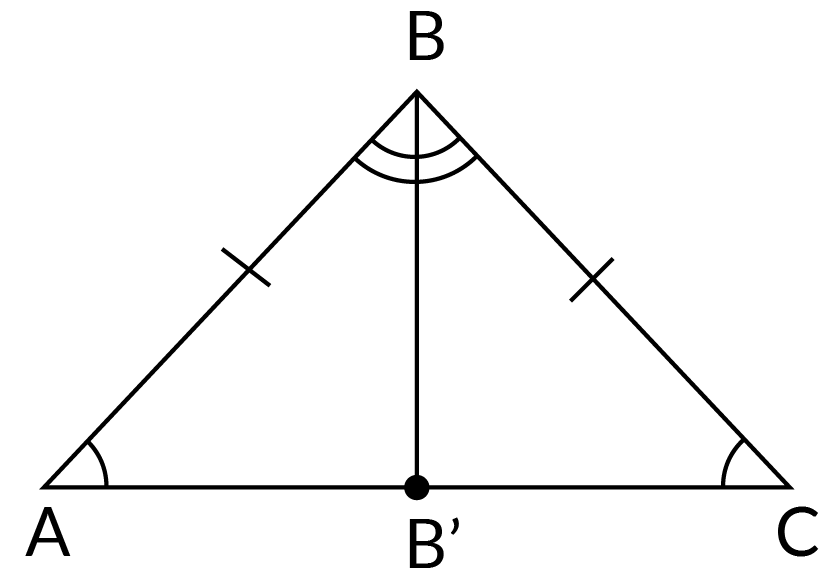Statement Reason
In $$\triangle \text{ABC},$$
$$\text{AB} = \text{CD}$$
Given, $$\because \triangle\, \rm{ABC}$$ is isosceles
$$\angle \text{ABB}’ = \angle \text{CBB}’$$  $$\because \text{BB}’$$ is an angle bisector
$$\text{BB}’ = \text{BB}’$$  Common side
$$\because \triangle \text{ABB}’ ⩭ \triangle\text{CBB}’$$  By SAS Postulate
$$\text{AB}’ = \text{CB}’$$  CPCTC (Congruent Parts of a Congruent Triangle are Congruent)

Hence $$△\rm{ABC}$$ and $$△\rm{ACD}$$ are proved to be congruent and $$\rm{AB}’ = \rm{CB}’$$.

## Exercise Questions

1. Prove that the two triangles are congruent.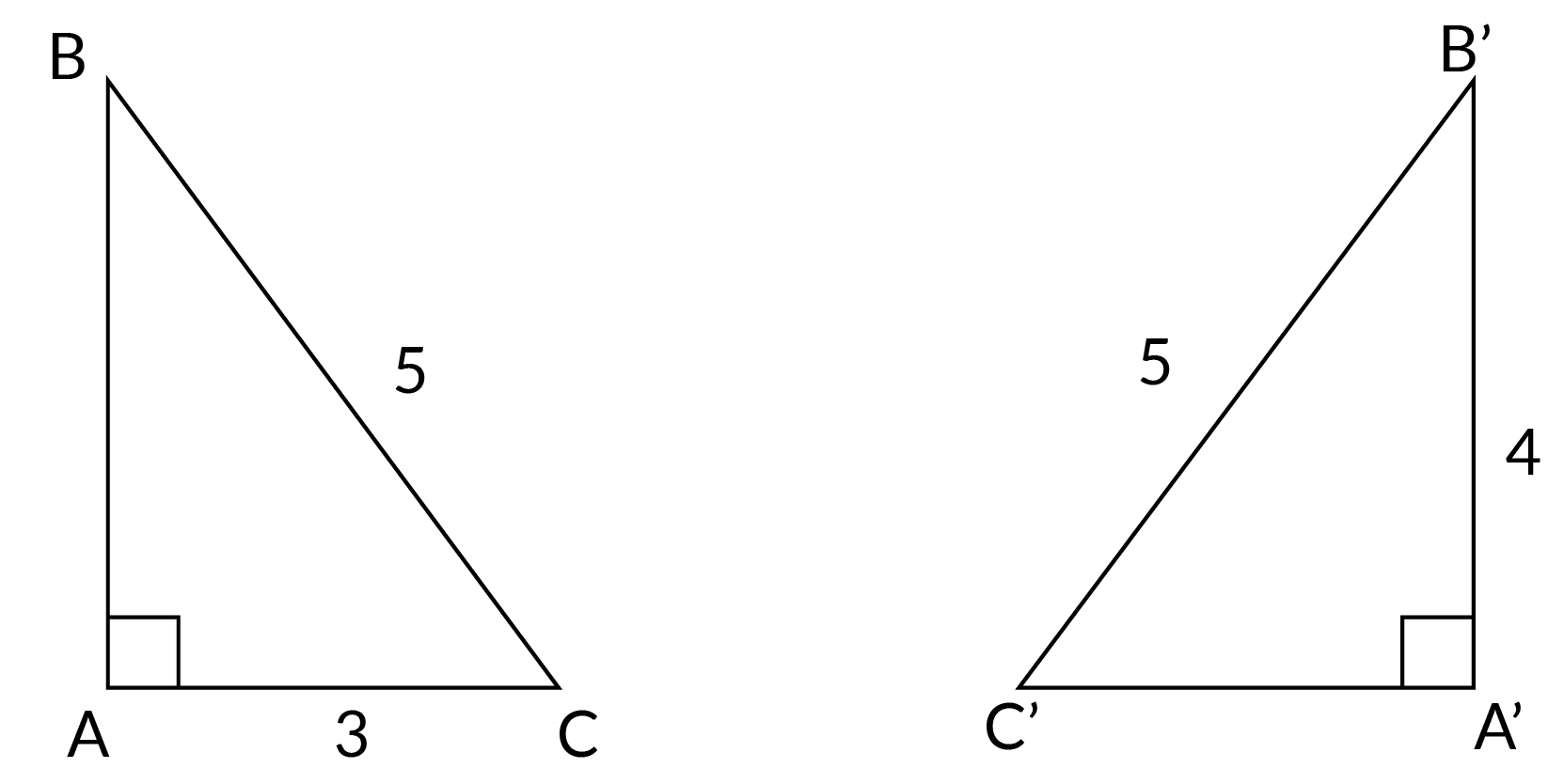2. Find the length of side $$\rm{AC},$$ if we know that $$\rm{QR} = 5$$.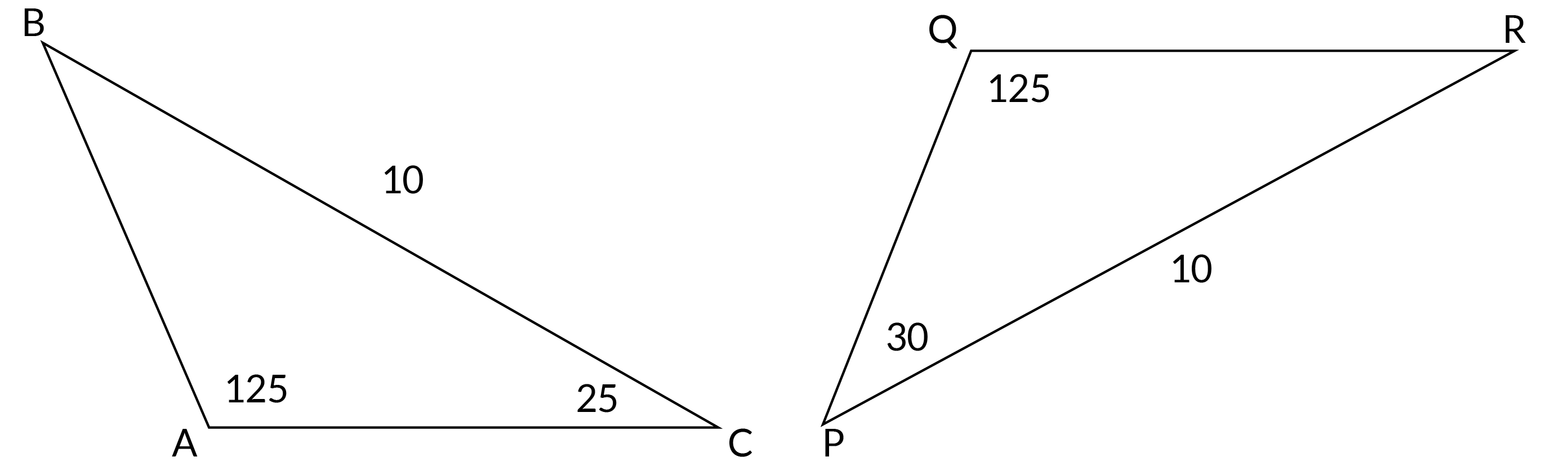3. $$\rm{M}$$ is the point of the $$\rm{AC}.$$ $$\rm{AI}$$ and $$\rm{CJ}$$ are perpendicular $$\rm{BM.}$$ Prove that triangles $$\rm{AIM}$$ and $$\rm{CJM}$$ are congruent.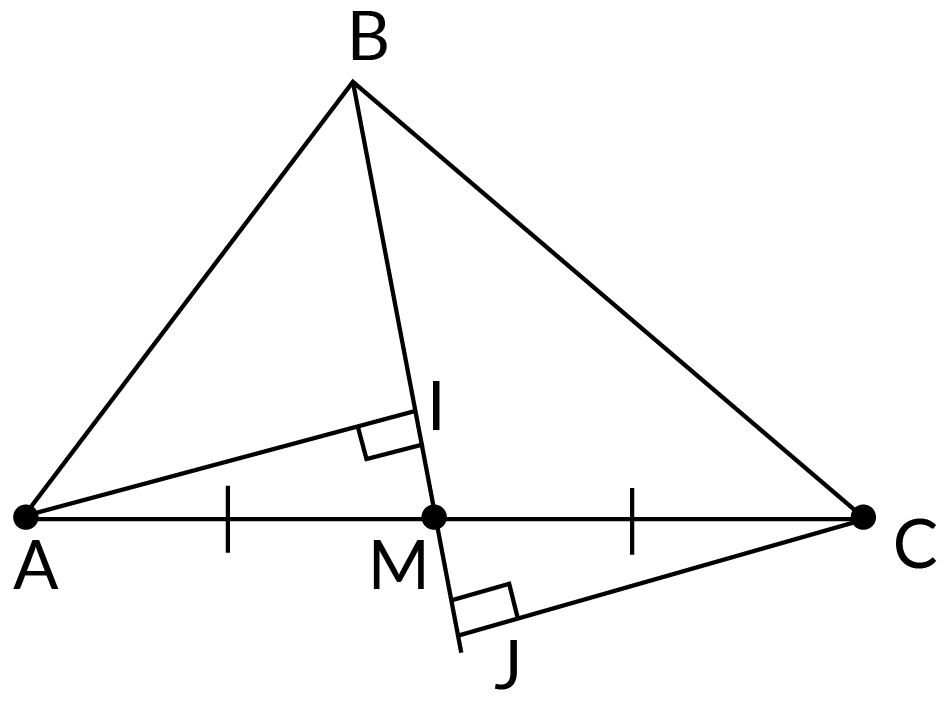## Summary

This blog discussed the congruency of triangles and the various postulates that can be used to prove congruency. It also discusses the CPCTC theorem, to draw further conclusions from congruency. A few examples were shown for a better understanding.

Cuemath, a student-friendly mathematics and coding platform, conducts regular Online Live Classes for academics and skill-development and their Mental Math App, on both iOS and Android, is a one-stop solution for kids to develop multiple skills. You can book a Free Class here and know more about the pricing and fees from Cuemath fee for all grades.

## What does congruent mean?

Congruent can be explained as agreeing or corresponding.

## What are congruent triangles?

Two triangles with $$3$$ equal sides and $$3$$ equal angles are said to be congruent with one another. They can be superimposed on one other with each and each side vertex coinciding to the other triangle

## When is RHS Test Applicable?

RHS test is only applicable on Right-angled triangles

## What does CPCTC mean?

CPCTC is the theorem that states Congruent Parts of a Congruent Triangle are Congruent.

## What is the SAS triangle Postulate?

If two triangles have one angle equal, and two sides on either side of the angle equal, the triangles are congruent by SAS Postulate.

## What is the AAS triangle Postulate?

Two equal angles and a side that does not lie between the two angles, prove that a pair of triangles are congruent by the AAS Postulate.

## What is the ASA triangle Postulate?

When two angles and a side between the two angles are equal, for $$2$$ triangles, they are said to be congruent by the ASA postulate.

## What are the 5 theorems of Congruency?

The 5 postulates to prove congruency are:

1. SSS triangle postulate

2. ASA triangle postulate

3. AAS triangle postulate

4. SAS triangle postulate

5. RHS triangle postulate

## External References

GIVE YOUR CHILD THE CUEMATH EDGE
Access Personalised Math learning through interactive worksheets, gamified concepts and grade-wise courses